# Simulation Examples¶

In this section, we use the `mrsimulator` tools to create spin systems and simulate spectrum with practical/experimental applications. The examples illustrate

• building spin systems (uncoupled and weakly-coupled),

• building NMR methods,

• simulating spectrum, and

For mrsimulator applications related to least-squares fitting, see the Fitting Examples (Least Squares).

## 1D NMR simulation (small molecules/crystalline solids)¶

The following examples are the NMR spectrum simulation of small molecules and crystalline solids for the following methods: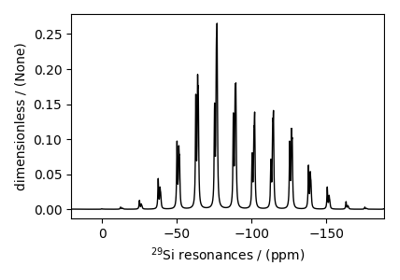Figure 26 Wollastonite, ²⁹Si (I=1/2)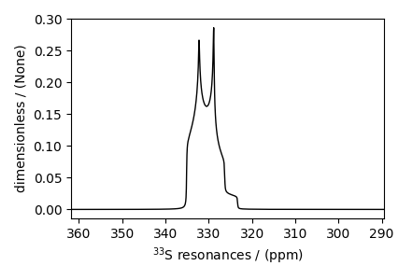Figure 27 Potassium Sulfate, ³³S (I=3/2)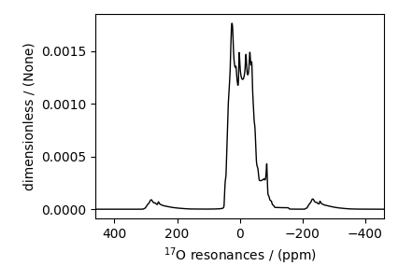Figure 28 Coesite, ¹⁷O (I=5/2)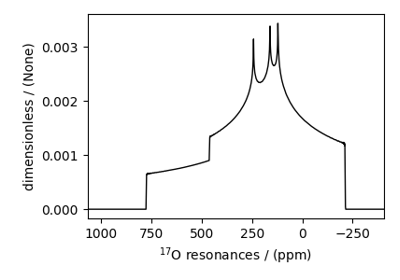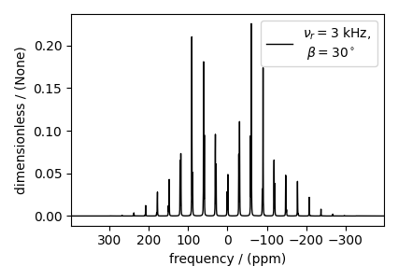Figure 35 Ethanol Revisited (¹H and ¹³C NMR)

## 1D NMR simulation (macromolecules/amorphous solids)¶

The following examples are the NMR spectrum simulation of macromolecules and amorphous materials for the following methods:

For NMR simulation of amorphous solids, we also show examples of simulating spectrum using user-defined model or using commonly accepted models such as Czjzek or extended Czjzek distribution.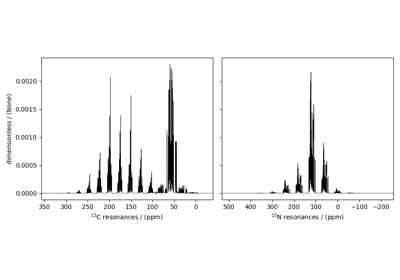Figure 39 Protein GB1, ¹³C and ¹⁵N (I=1/2)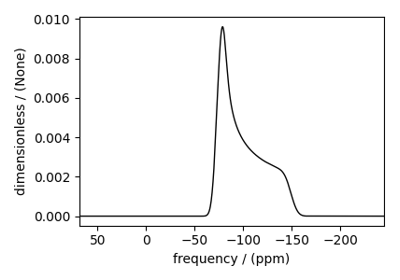Figure 40 Amorphous material, ²⁹Si (I=1/2)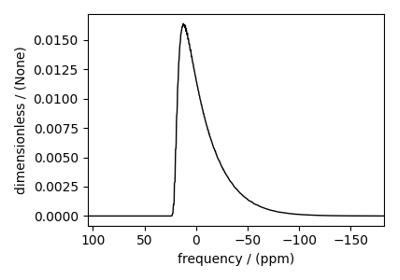Figure 41 Amorphous material, ²⁷Al (I=5/2)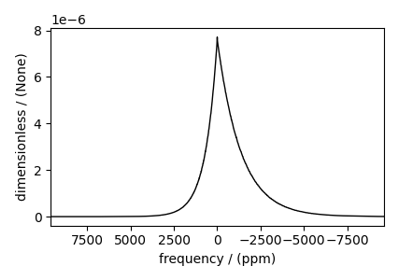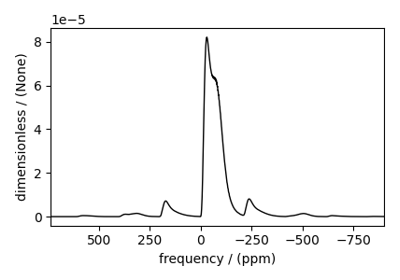## 2D NMR simulation (Crystalline solids)¶

The following examples are the NMR spectrum simulation for crystalline solids. The examples include the illustrations for the following methods: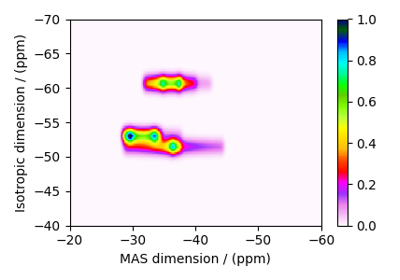Figure 44 RbNO₃, ⁸⁷Rb (I=3/2) 3QMAS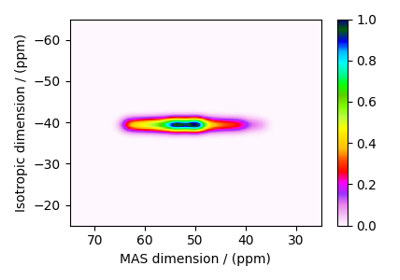Figure 45 Albite, ²⁷Al (I=5/2) 3QMAS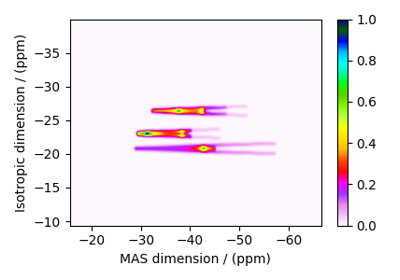Figure 46 RbNO₃, ⁸⁷Rb (I=3/2) STMAS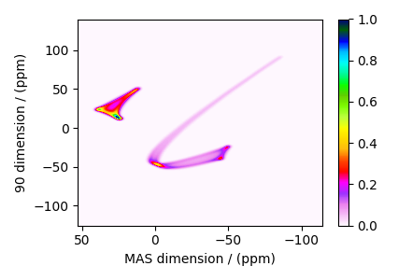Figure 47 Rb₂SO₄, ⁸⁷Rb (I=3/2) SAS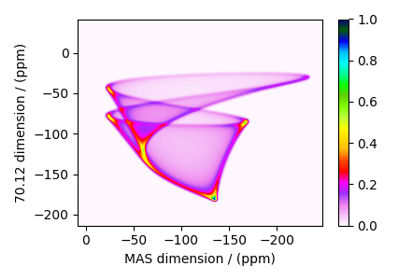Figure 48 Rb₂CrO₄, ⁸⁷Rb (I=3/2) SAS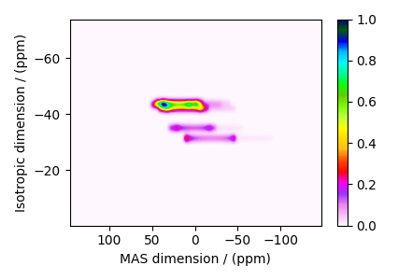Figure 49 Coesite, ¹⁷O (I=5/2) 3QMAS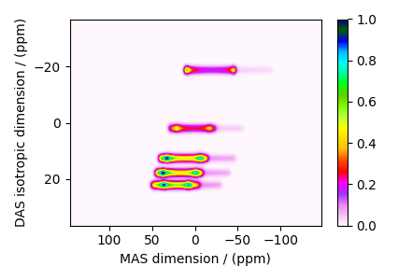Figure 50 Coesite, ¹⁷O (I=5/2) DAS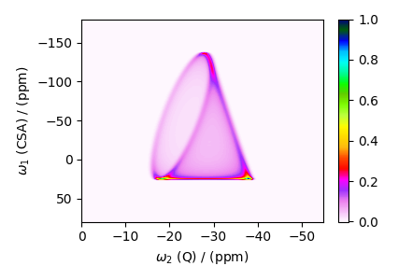Figure 51 Rb₂CrO₄, ⁸⁷Rb (I=3/2) COASTER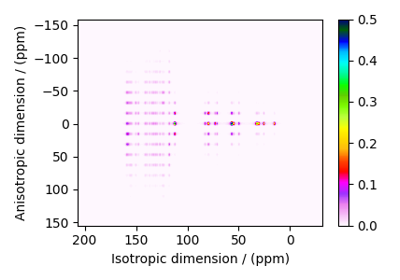Figure 52 Itraconazole, ¹³C (I=1/2) PASS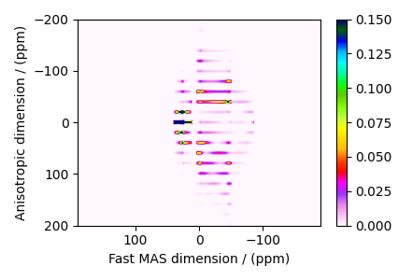Figure 53 Rb₂SO₄, ⁸⁷Rb (I=3/2) QMAT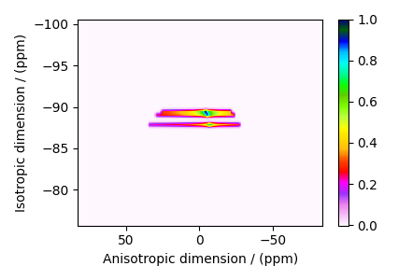Figure 54 Wollastonite, ²⁹Si (I=1/2), MAF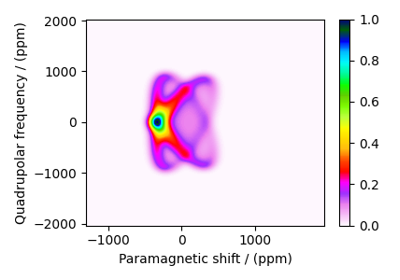Figure 55 MCl₂.2D₂O, ²H (I=1) Shifting-d echo

## 2D NMR simulation (Disordered/Amorphous solids)¶

The following examples are the NMR spectrum simulation for amorphous solids. The examples include the illustrations for the following methods: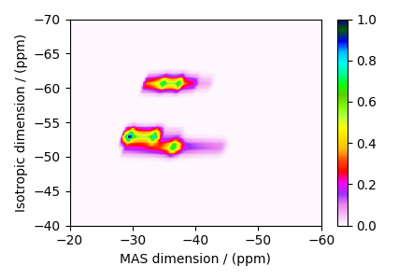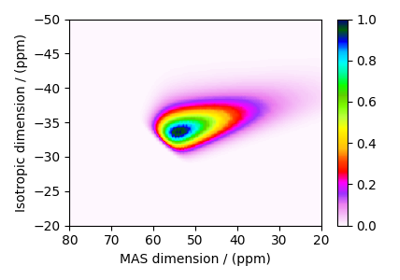Gallery generated by Sphinx-Gallery